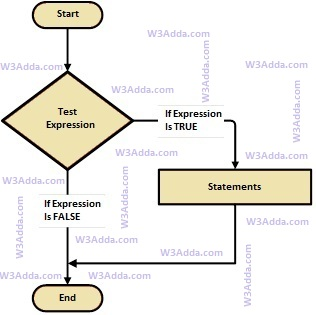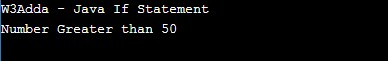# Java If Statement

In this tutorial you will learn about the Java If Statement and its application with practical example.

## Java if statement

Java if statement allows a block of code to be executed only when a specified condition is true. An if statement evaluates a boolean expression followed by one or more statements. The given boolean expression results in a boolean value that can only be either true or false.

## Java If Statement Flow Diagramjava-if-statement

Syntax:-

Here, a condition is a Boolean expression that results in either True or False, if it results in True then statements inside the body is executed, if it results in False then execution is skipped from if body.

Example:-

Output:-In this tutorial we have learn about the Java If Statement and its application with practical example. I hope you will like this tutorial.